# keeping last duplicate in numbered listhi community,

transactions is a numbered list and module has 3 line items, 2 are text and 1 is number formatted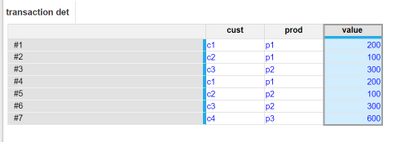so the ask is to find duplicate combination of customer, prod and value in the list and in case of match , keep the last item and delete the first item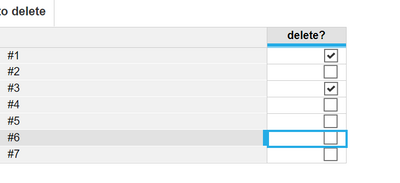here, 1 and 4 are duplicate , 3 and 6 are duplicate , but we need to keep 4 and 6 and delete 1 and 3 respectively,

any help will be appreciated

Tagged:

•Guessing the answer would be no, its always worth asking if we could bring the data into Anaplan the way that we want it.

If not, then I think there are a couple of ways to achieve this. Here is one of those examples:

in your 'transaction det' module I would create a line that concatenates the cust, prod, and value. Then we will create a new list and import the first occurrence of each into that list. Then we want to get a line item that has our new list. In my example I am doing this in my Concat List line item: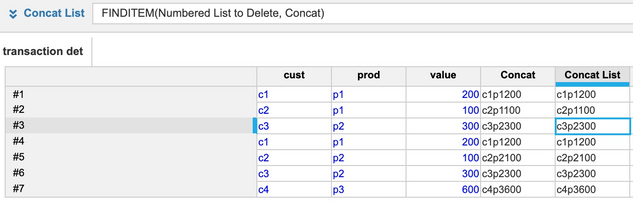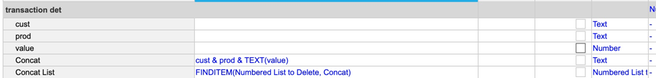From there we need to find the maximum number of occurrences that each unique concatenation has.

I have done this in 2 steps:

Cumulate: CUMULATE(1, FALSE, Numbered List)  This formula is just counting the total number of items within the list and counting from 1 to however many items you have.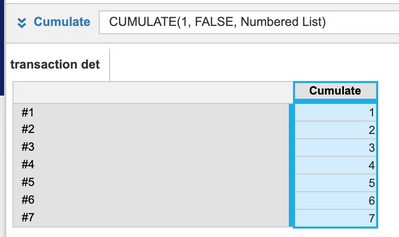And secondly, we find the max number of the cumulate against our concatenated list dimension that we created earlier.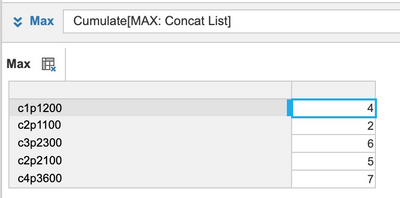Now that we know the Max of each unique concatenation, then we can compare the cumulate line to the max line to determine what we should delete.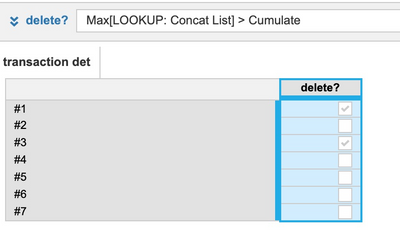There may be a more efficient solution out there in terms of calculations/being less process heavy, but the is one option that would get the job done.

Jason

•Below is the quick solution for the same

Created the module same Transaction Det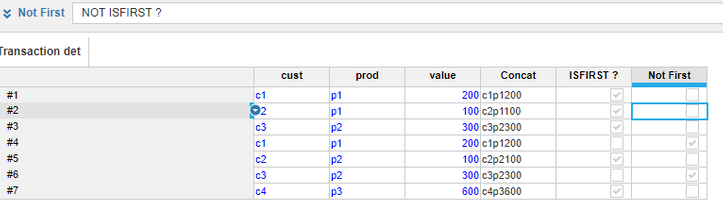Additionally Created Three line item :-

First Line item to concatenate the data for which we want to make the check.

Second Boolean to check if it is the First Occurrence in the Data

Third Line item Not First Occurrence which you can use to delete the numbered list.

Below is the Blueprint of the same.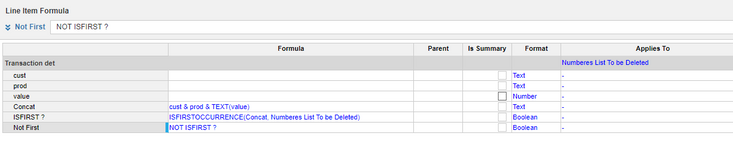Thanks,

N

•I thought about doing the same, but I got stuck on the point that there could probably be more than 2 occurrences of the same concatenation. If there were 3 occurences then we would want to delete the first 2, and by using the first occurrence then we would only delete 1. At least this was my assumption. If we can assume that we are adding one record each time the import is run, then I agree that your solution is much faster and easier!! If it is possible that more than one record gets imported with multiple instances of the same concatenation then the delete action would need to get ran an undefined number of times to clear all of the not first occurrences until we get to where the is only one of each remaining, or you would need to use a different solution that could clear them all at once.

Jason

•so this is  giving the latest duplicate to be deleted i.e 4 and 6, but we want 1 and 3 to be deleted, this was the first thing i thought of ,

thanks

•hi @jasonblinn ,

thanks for the solution

just one thing , so you created a separate list of all the unique concatenations and in the main module, you found max position of each unique concatenation , will these work in case of if here are more than 2 duplicates?

•Yes, since the cumulate will continually count (Up to 50 million) then when there are more than two transactions that have the same concatenation then it would remove all except the last one.

Using the same example, I just added lines 8-11 and copied the cust, prod and value from #1. As you can see, all of the transactions that match this data except for #11 have been flagged for delete (#1, #4, #8, #9, #10):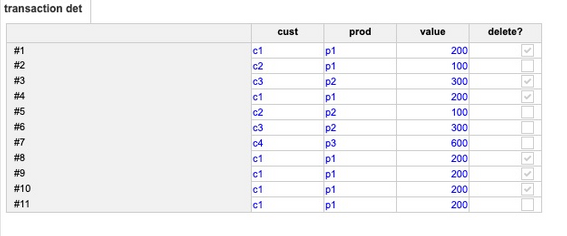Jason

•This is great,

Thanks a lot

•Thanks for the solution,

Actually  it was given to us as a poc to do and in actual requirement, it was on base of these properties and date of loading the transactions, so yes date of loading is recorded and in case multiple duplicates are loaded in that same date, we had to to try to keep the last added item and delete early entries, not on time but on date basis

•Very Useful.